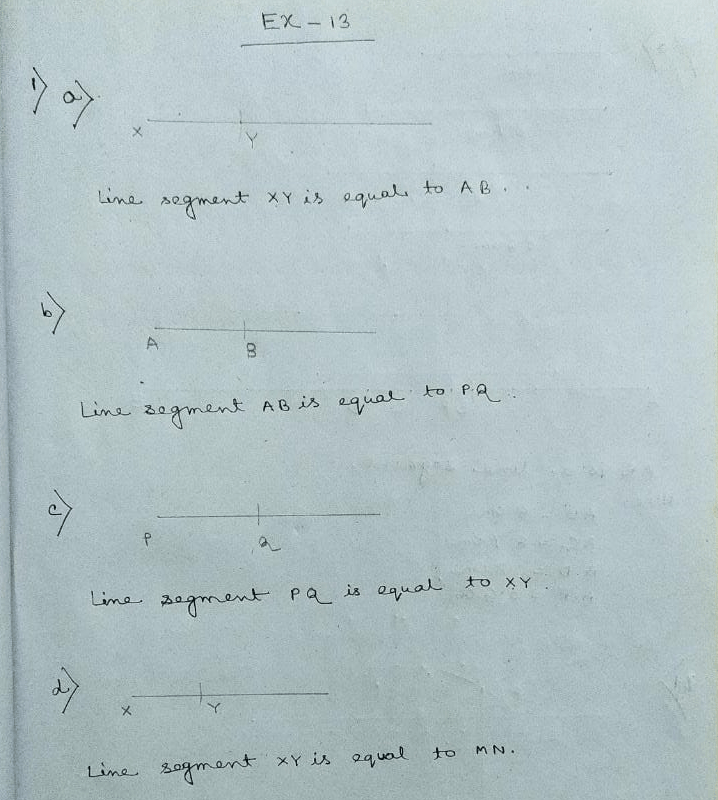# New Learning Composite Mathematics Class 6 SK Gupta Anubhuti Gangal Basic Constructions Chapter 13 Solution

## New Learning Composite Mathematics Class 6 SK Gupta Anubhuti Gangal Basic Constructions Chapter 13 SolutionProcess:

(a) At first I draw a ray BC. Write B as centre and any convenient radius I draw an arc, cutting BC at Q. With Q as center and same radius I draw another arc to cut the first arc at P. Now I join BP. Thus ∠PBQ = 600.

Then with compass I bisect ∠PBQ, BO is the angel bisector.

Thus ∠ABO = ∠OBC = 300

(b) At first I draw a ray OR. Then I construct an angel ∠PQR = 900. Then with compass I bisect ∠PQR, QS is the angel bisector.

Thus ∠PQS = ∠SQR = 450Process:

(c) At first I draw a line AC. With a vertex B or AC. I construct ∠FBC = 1500.

Then with compass I bisect ∠FBC, BD is the angel bisector.

Thus ∠FBD = ∠DBC = 750

(d) At first I draw a line AC. With a vertex B on AC, I construct ∠FBC = 1350

Then with compass I bisect ∠FBC, BD is the angel bisector.

Thus ∠FBD = ∠DBC = 67 1/20Process:

(e) I draw a line AC, with point B on the AC as vertex, construct ∠CBD = 900. Then ∠ABD = 900

Then, ∠CBE = 900 + 450 = 1350

(f) I draw a line AC. With a vertex B on , I construct ∠CBD = 1200.

Then, I bisect ∠ABD, BE is the angel bisector

Thus, ∠EBC = 1200 + 300 = 1500

(g) I draw a line AC with B as centre and any convenient radius I draw an arc to cut the BC at X with X as centre and the radius, I draw another arc to cut the first arc at Y. with Y as centre and the same radius, I draw another arc to cut the another arc at Z. Then I draw the ray BD through B and Z. Thus ∠CBD = 1200Updated: June 19, 2021 — 3:14 pm